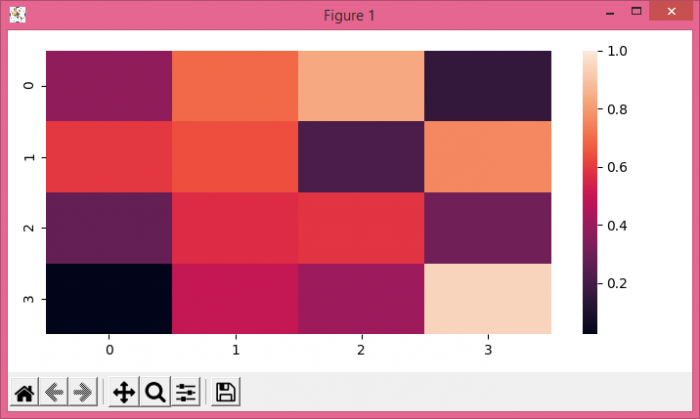# Set Max value for color bar on Seaborn heatmap using Matplotlib

To set a value for color bar on Seaborn heatmap, we can take following Steps−

• Create random data using numpy.
• Use heatmap() method to plot rectangular data as a color-encoded matrix.
• To display the figure, use show() method.

## Example

import numpy as np
import seaborn as sns
from matplotlib import pyplot as plt
plt.rcParams["figure.figsize"] = [7.00, 3.50]
plt.rcParams["figure.autolayout"] = True
data = np.random.rand(4, 4)
ax = sns.heatmap(data, vmax=1)
plt.show()

## Output